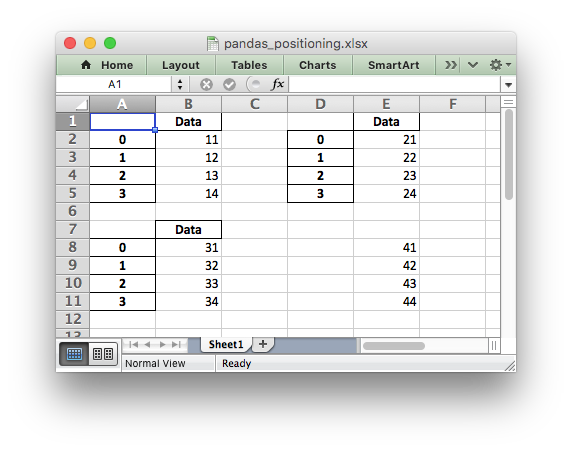# Example: Pandas Excel dataframe positioning#

An example of positioning dataframes in a worksheet using Pandas and XlsxWriter. It also demonstrates how to write a dataframe without the header and index.```##############################################################################
#
# An example of positioning dataframes in a worksheet using Pandas and
# XlsxWriter.
#
# Copyright 2013-2023, John McNamara, jmcnamara@cpan.org
#

import pandas as pd

# Create some Pandas dataframes from some data.
df1 = pd.DataFrame({"Data": [11, 12, 13, 14]})
df2 = pd.DataFrame({"Data": [21, 22, 23, 24]})
df3 = pd.DataFrame({"Data": [31, 32, 33, 34]})
df4 = pd.DataFrame({"Data": [41, 42, 43, 44]})

# Create a Pandas Excel writer using XlsxWriter as the engine.
writer = pd.ExcelWriter("pandas_positioning.xlsx", engine="xlsxwriter")

# Position the dataframes in the worksheet.
df1.to_excel(writer, sheet_name="Sheet1")  # Default position, cell A1.
df2.to_excel(writer, sheet_name="Sheet1", startcol=3)
df3.to_excel(writer, sheet_name="Sheet1", startrow=6)

# It is also possible to write the dataframe without the header and index.
df4.to_excel(
writer, sheet_name="Sheet1", startrow=7, startcol=4, header=False, index=False
)

# Close the Pandas Excel writer and output the Excel file.
writer.close()
```